Evaluating Summation Notation (Function Mode)
 General summations:

A shorthand notation is used to represent sums with more than a few terms.  This shorthand notation uses the Greek letter sigma (which denotes sum).  If we have a rule such as f (n) which assigns the value f (n) to each integer n in the set of integers {j, j+1, j+2, ... k}, we can represent the sum as: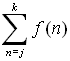=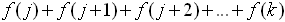On the graphing calculator:

We will be using the sum feature and the seq feature to work with a general summation.
On the calculator, we will interpret a summation as follows: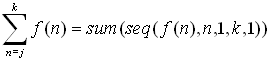2nd STAT (LIST) → MATH #5 sum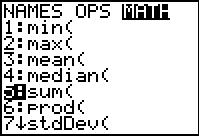The format for sum is  sum( list )
where list will be the terms of the rule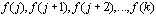.

2nd STAT (LIST) OPS  Choose #5 seq(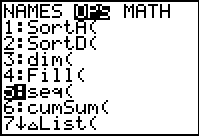The format for seq:  expression, variable,
starting value, ending value, increment.

While sequences have a domain of natural numbers, {1, 2, 3, 4, ...}, in Func mode, the sequence command will accept integer domain values less than one.  (In Seq mode, however, the sequence command will accept only integer domain values of 1 or greater.)

 Example 1:  Evaluate: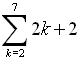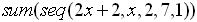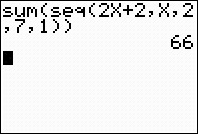notice that this sum starts with k = 2
 Example 2:    Evaluate: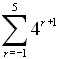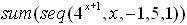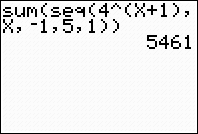(For ease of entry, X was used as the variable in both examples above.
If you wish to use the designated variable, type it into the calculator using the alpha key.)

If you think you are going to forget these commands:Another approach to Example 1: Type the number of terms needed into L1. Type the rule into L2. Add the answers manually on the HOME screen.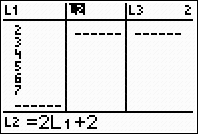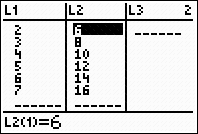Or, have the calculator do the adding.  If you do not want to add manually on the home
screen, you can use the cumulative summation feature (cumSum) to add L2.

Remember that Example 1 starts with k = 2.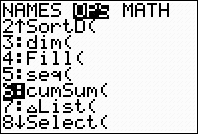To find cumSum( , go to LIST (2nd STAT), arrow to OPS heading, and choose #6 cumSum(.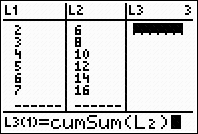Enter cumSum(L2) as the pattern to determine L3.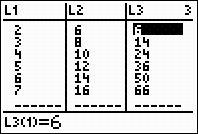The entry in column L3 corresponding to the value 7 in L1 will be the sum.

 Summations and Sequences:

Sums, such as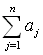, where j = 1, may represent the summation of a sequence, called a series.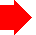Remember, sequences have a domain of natural numbers, {1, 2, 3, 4, ...}. In the sequence related problems below, the starting value in the summation will be greater than or equal to 1.
 Again, we will be using the sum and seq features of the calculator to find the summations.  The summation is interpreted as: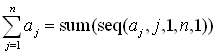2nd STAT (LIST) → MATH #5 sum2nd STAT (LIST) → OPS Choose #5 seq(Example 3:            Evaluate: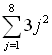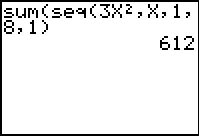This example uses x as the variable, instead of using j.  The x is simply easier to enter into the calculator. Example 4:        Evaluate: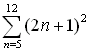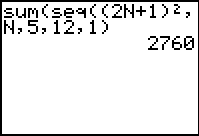This example uses n as the variable -- obtained by engaging the alpha key.Finding Your Way Around TABLE of  CONTENTS